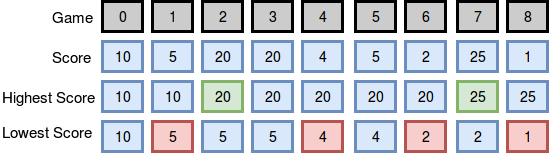Breaking the Records, Algorithm problem

# Breaking the Records, Algorithm problem

Maria plays n  games of college basketball in a season. Because she wants to go pro, she tracks her points scored per game sequentially in an array defined as score = [s0,s1…sn-1]. After each game i , she checks to see if score breaks her record for most or least points scored si so far during that season.

Given Maria’s array of for a season of games, find and print the number of times she breaks her record for most and least points scored during the season.

Note: Assume her records for most and least points at the start of the season are the number of points scored during the first game of the season.

Input Format

The first line contains an integer denoting (the number of games).
The second line contains space-separated integers describing the respective values of .

Constraints

Output Format

Print two space-seperated integers describing the respective numbers of times her best (highest) score increased and her worst (lowest) score decreased.

Sample Input 0

9
10 5 20 20 4 5 2 25 1


Sample Output 0

2 4


Explanation 0

The diagram below depicts the number of times Maria broke her best and worst records throughout the season:She broke her best record twice (after games and ) and her worst record four times (after games , , , and ), so we print 2 4 as our answer. Note that she did not break her record for best score during game , as her score during that game was not strictly greater than her best record at the time.

Sample Input 1

10
3 4 21 36 10 28 35 5 24 42


Sample Output 1

4 0


Explanation 1

The diagram below depicts the number of times Maria broke her best and worst records throughout the season:She broke her best record four times (after games , , , and ) and her worst record zero times (no score during the season was lower than the one she earned during her first game), so we print 4 0 as our answer.

Solution in PHP

If any problem, feel free to comment or contact.


<?php

$handle = fopen ("php://stdin","r"); fscanf($handle,"%d",$n);$score_temp = fgets($handle);$score = explode(" ",$score_temp); array_walk($score,'intval');

$highest=0;$lowest=0;

$temp=$score;
$temp2=$score;
for ($i=0;$i < $n ;$i++) {
if ($temp<$score[$i]) {$temp=$score[$i];
$highest++; } if ($temp2>$score[$i]) {
$temp2=$score[$i];$lowest++;
}
}

echo $highest, " ",$lowest;

?>